# R package ggplotAssist

2017-10-24

``The 'ggplotAssist' is an RStudio addin for teaching and learning plot generation using the 'ggplot2' package. You can learn each steps of plot generation - aesthetics mapping, select geometries, add scales, apply theme - by clicking your mouse without coding. You can see the resultant plot and see the each steps of plot layer by layer. You get resultant code for ggplot. ``

## Prerequisite

You have to install the developmental version of R package `editData` from github.

``````#install.packages("devtools")
devtools::install_github("cardiomoon/editData")``````

## Install package

You can install `ggplotAssist` package from github.

``````#install.packages("devtools")
devtools::install_github("cardiomoon/ggplotAssist")``````

## Usage: As an RStudio Add-in

This addin can be used to interactively generate a `ggplot` using `ggplot2` package. The intended way to use this is as follows:

1. Highlight a symbol naming a `data.frame` or a `tibble` in your R session, e.g. `msleep`(1). Execute this addin(arrow), to interactively manipulate it.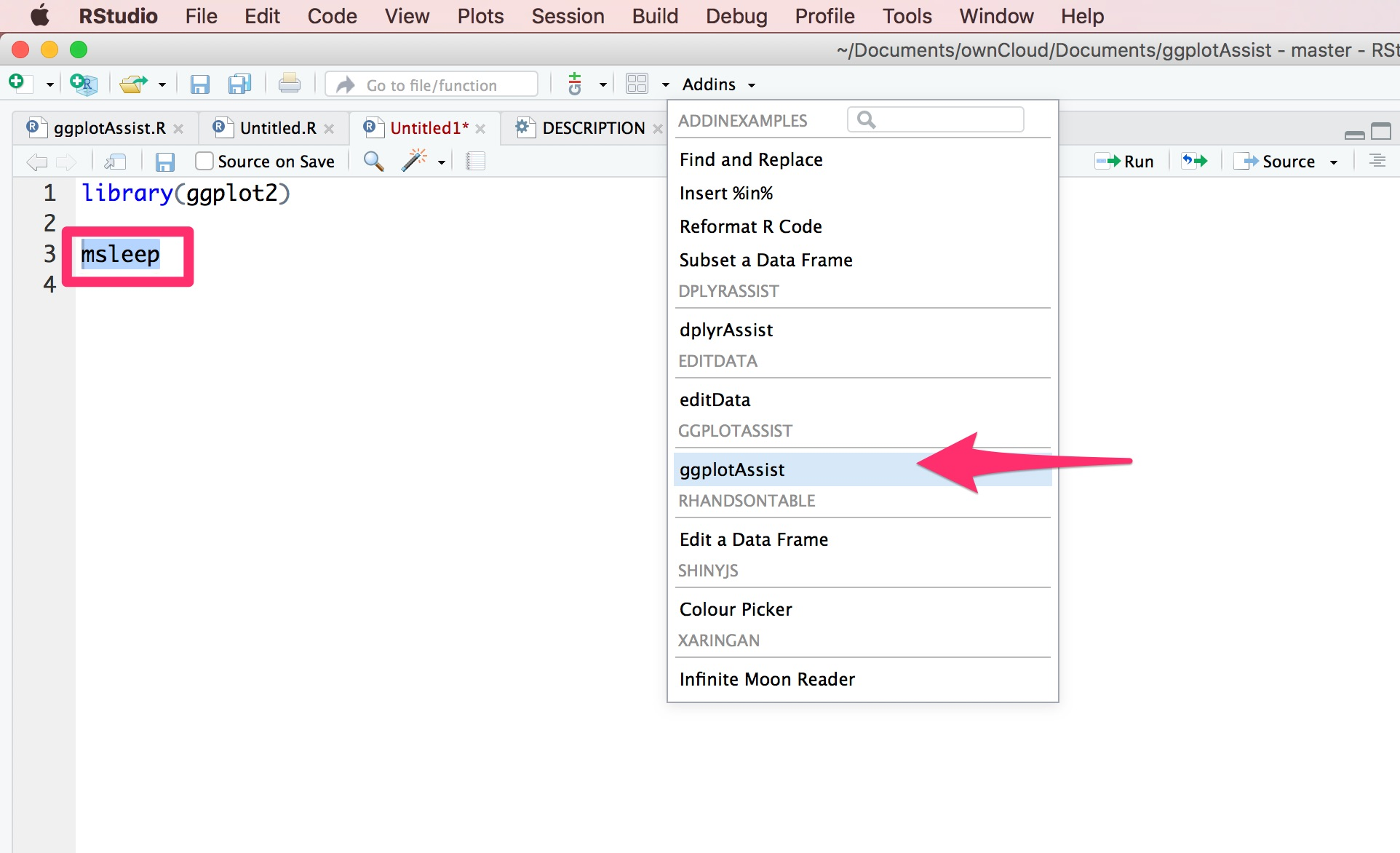1. You can see a brower window. You can see the data name(1) and R code for ggplot(2). Select `x`(3) and `bodywt`(4) to map `bodywt` as a x-axis variable.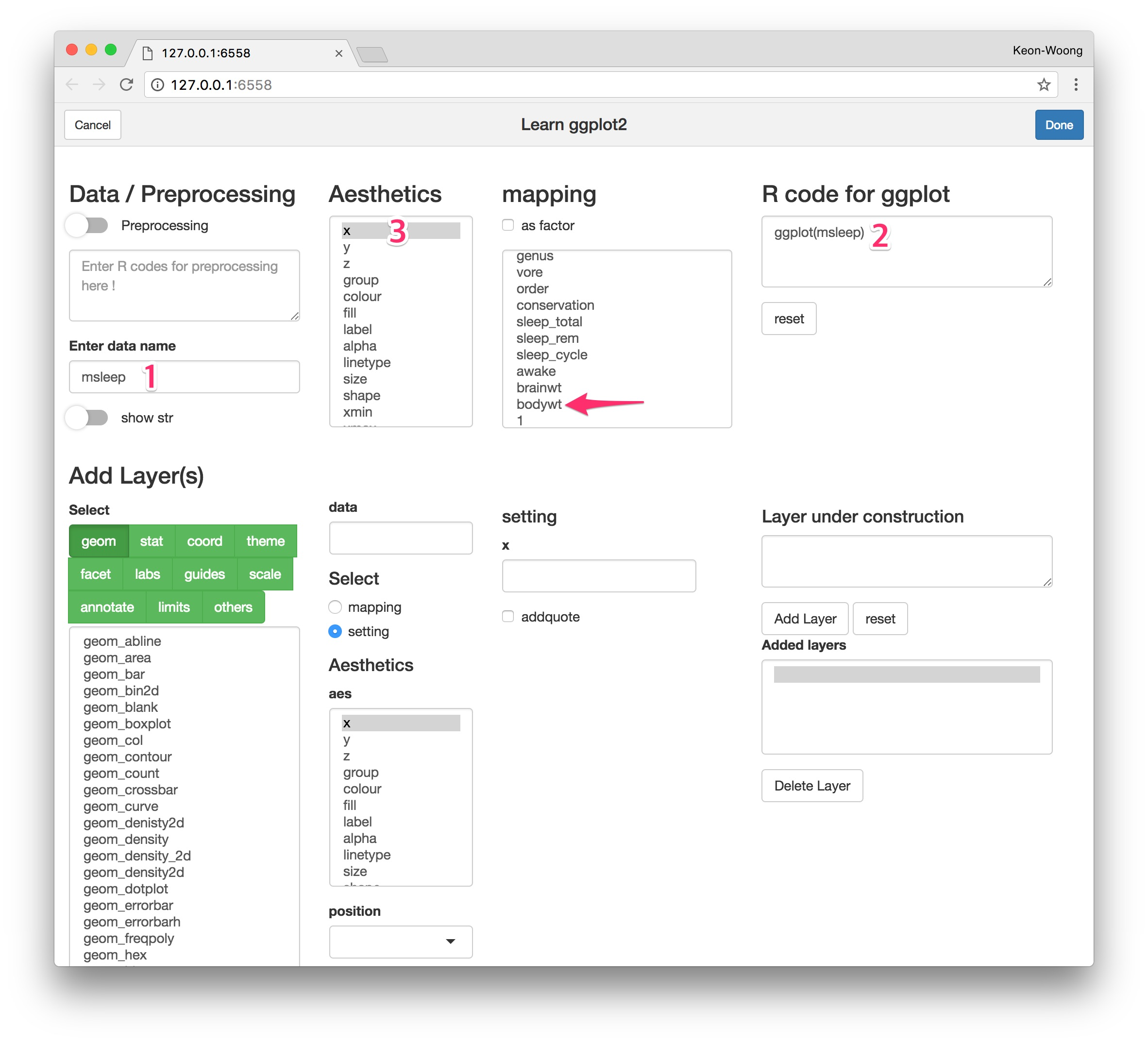1. You can see the R code for ggplot(1). Select `y` and `brainwt` to map the y-axis variable.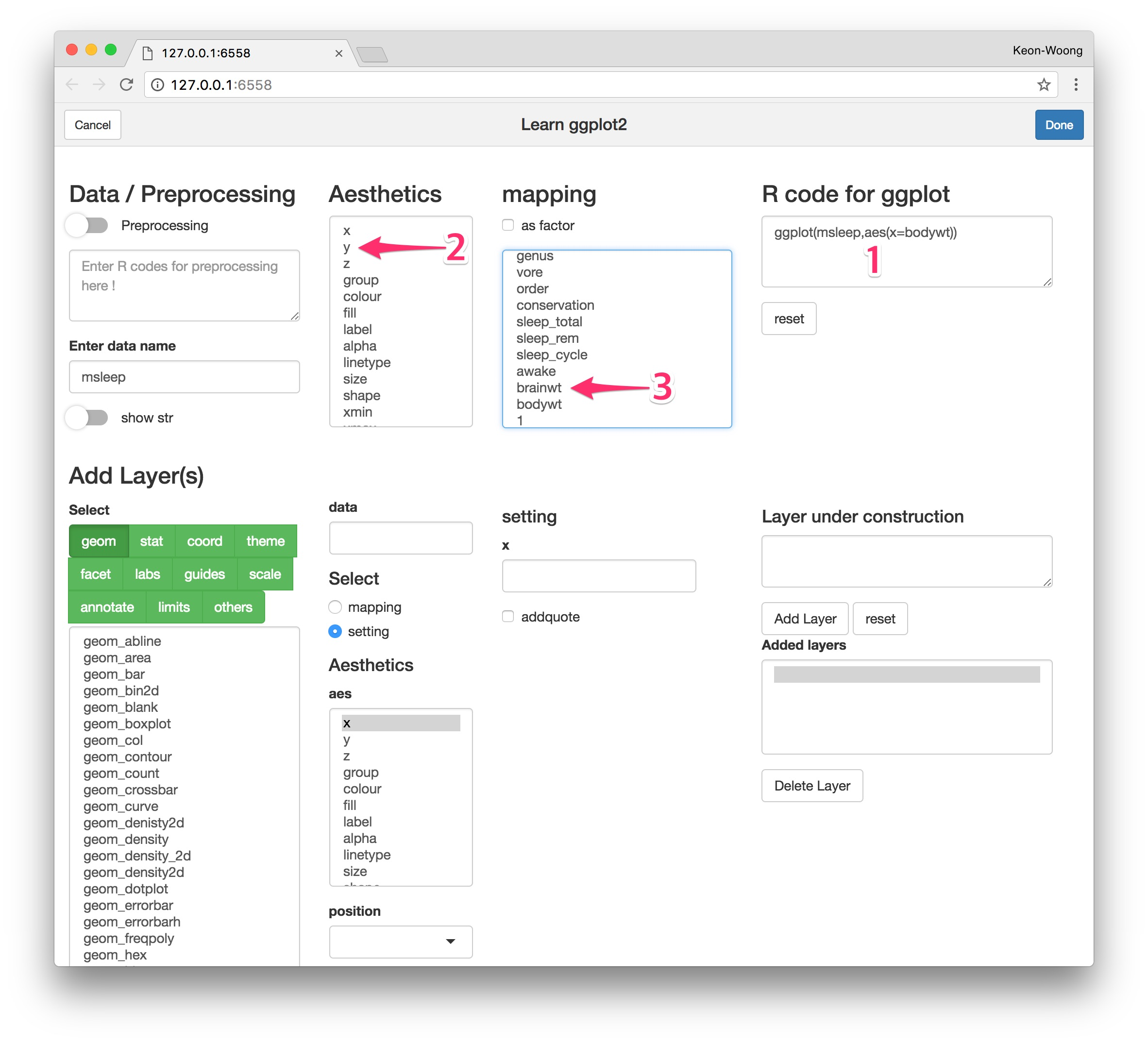1. To add geoms to ggplot, press `geom` button(1) and select `geom_point`(2). You can mapping or setting the aesthetics of geom_point. You can see the R code for this layer(3). In the lower part of window, you can see two R codes and two plots. In the lower left portion, you can see the R code for plot(4) and resultant plot(5). In the lower right portion, you can see the R code for plot with R code for layer under construction(6) and resultant plot(7). This is a plot preview.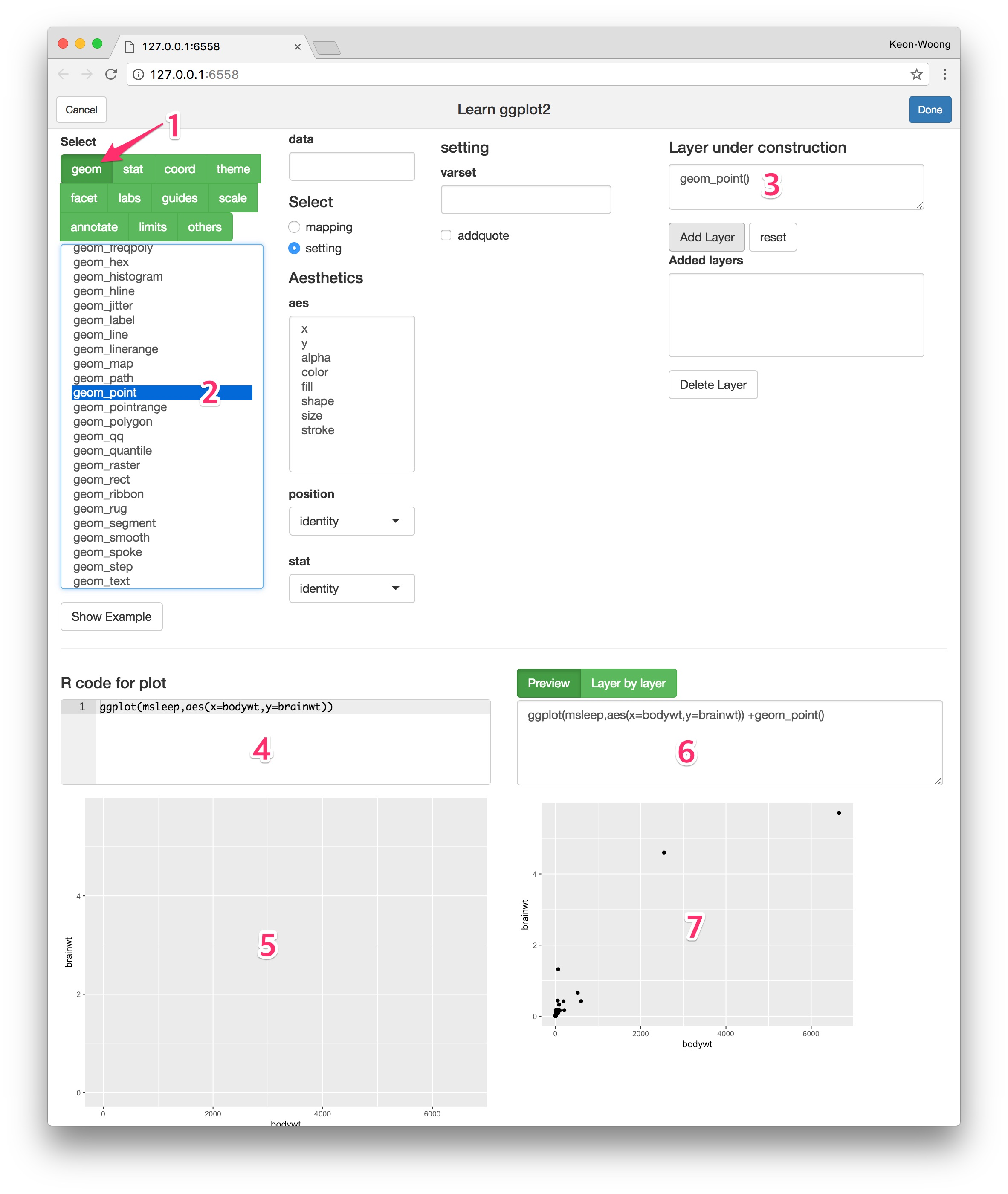1. If you finish to make a layer, press `Add Layer` button(1) to add the layer. You can see added layers(2). You can delete a layer with `delete layer` button after select a layer to delete.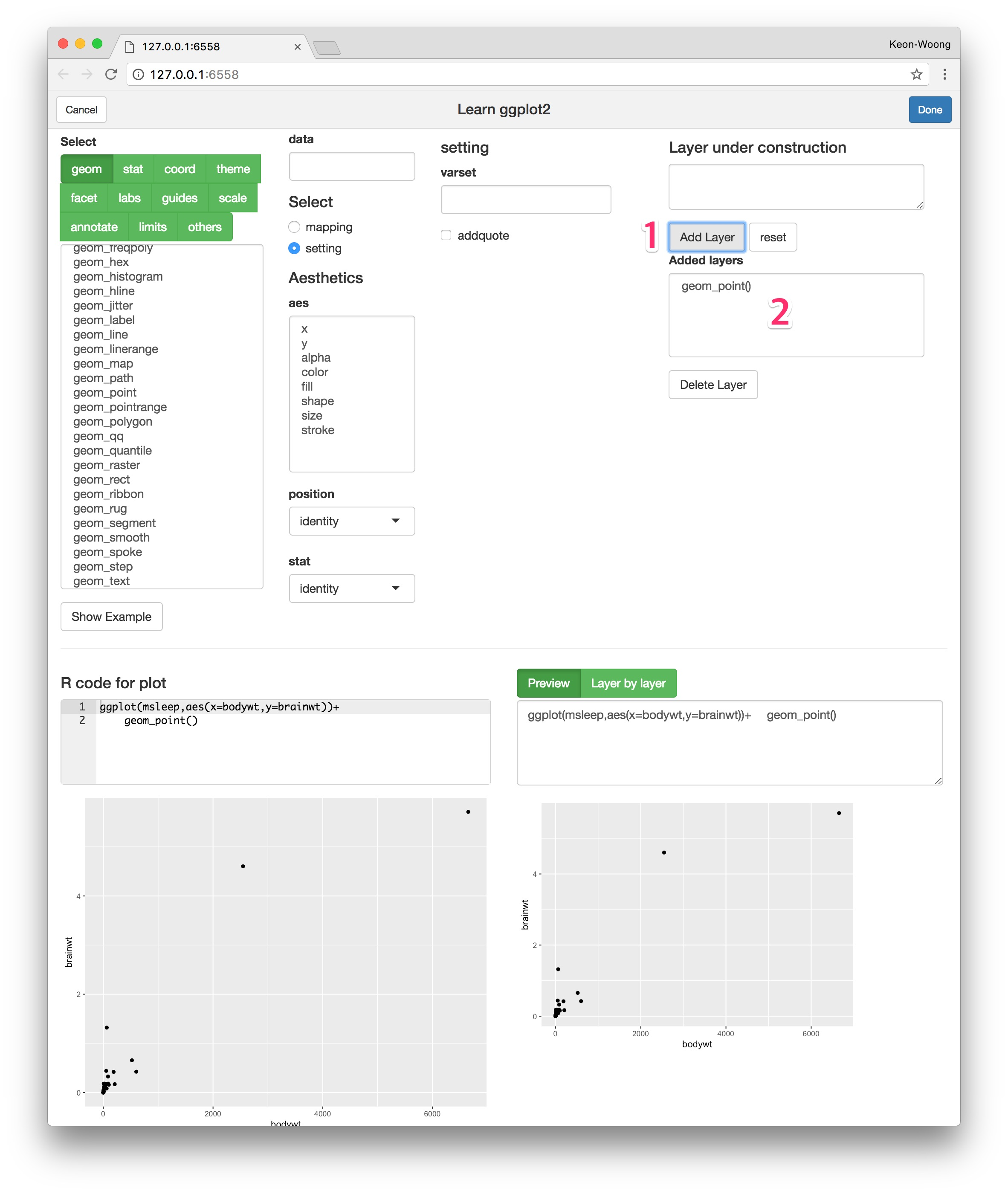1. To add theme, press `theme` button(1) and select `theme_bw`(2). You can see the code for theme_bw(3) and plot preview(4,5). Press `Add Layer` button(6) to add the code.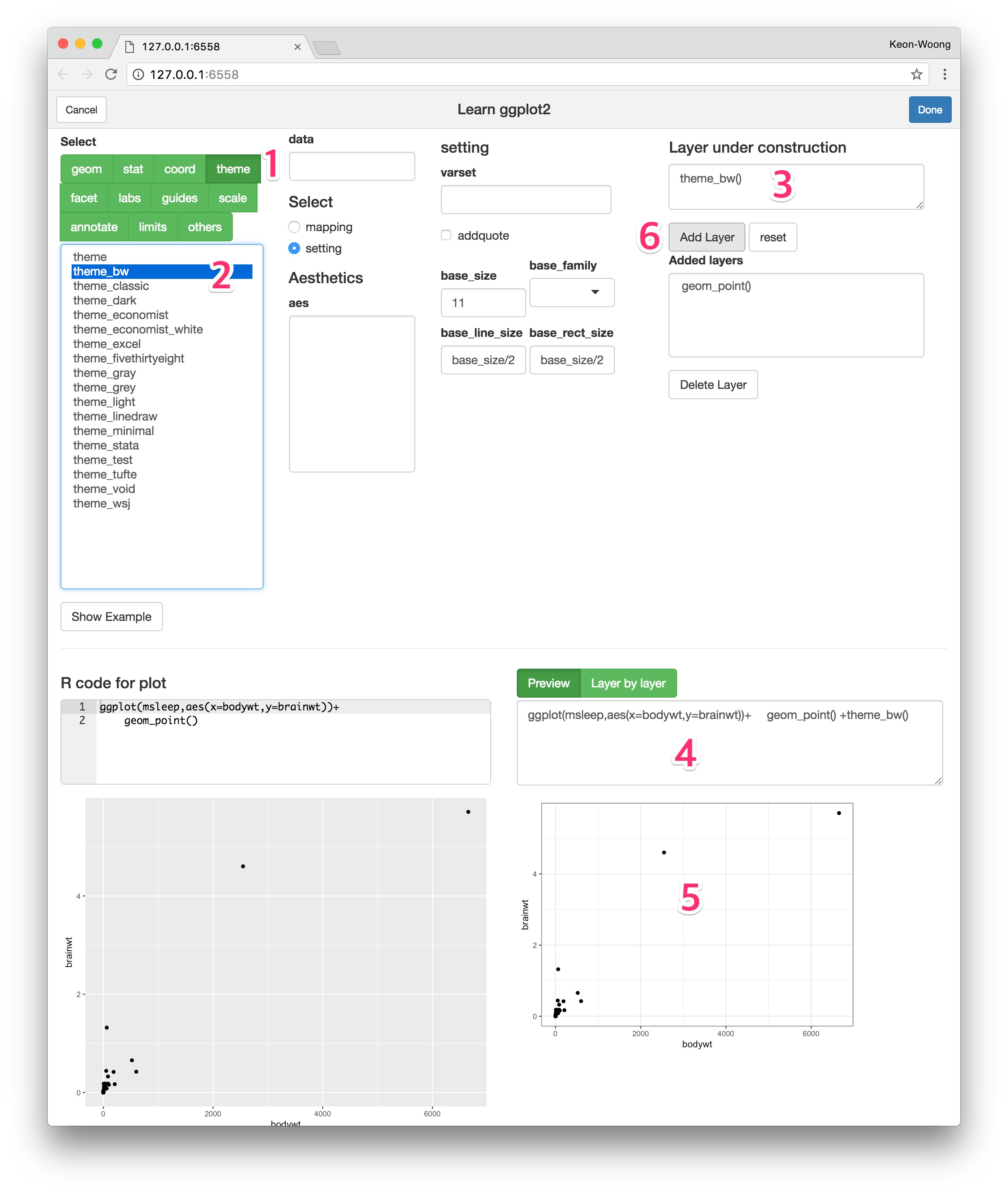2. To apply a log scale to x-axis, press `scale` button(1) and select `scale_x_log10` function(2). You can set the arguments of scale_x_log10() function(3). You can see the code for scale(4) and plot preview(5,6). To add math format to the log scale, press `Add math-format` button(9). You can repeat this step to apply a log scale to y-axis.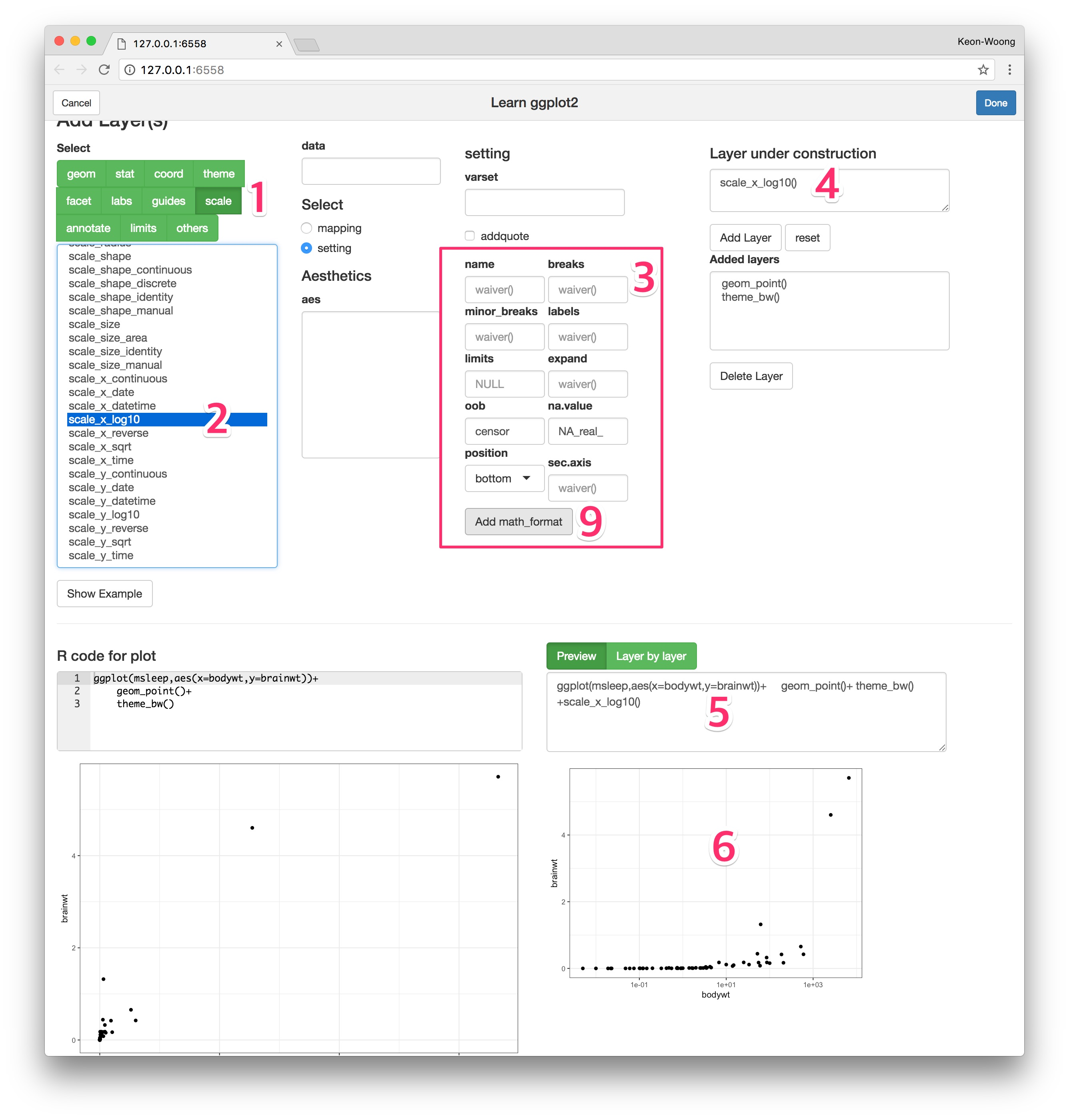3. You can add logticks to your plot. Press `annotate` button(1) and select annotation_logticks function(2). You can see the arguments and default values of this function(3). Set the side argument `trbl`(4). You can see the R code for this layer(5) and plot preview(6.7). Press `Add Layer` button(8) to add this layer.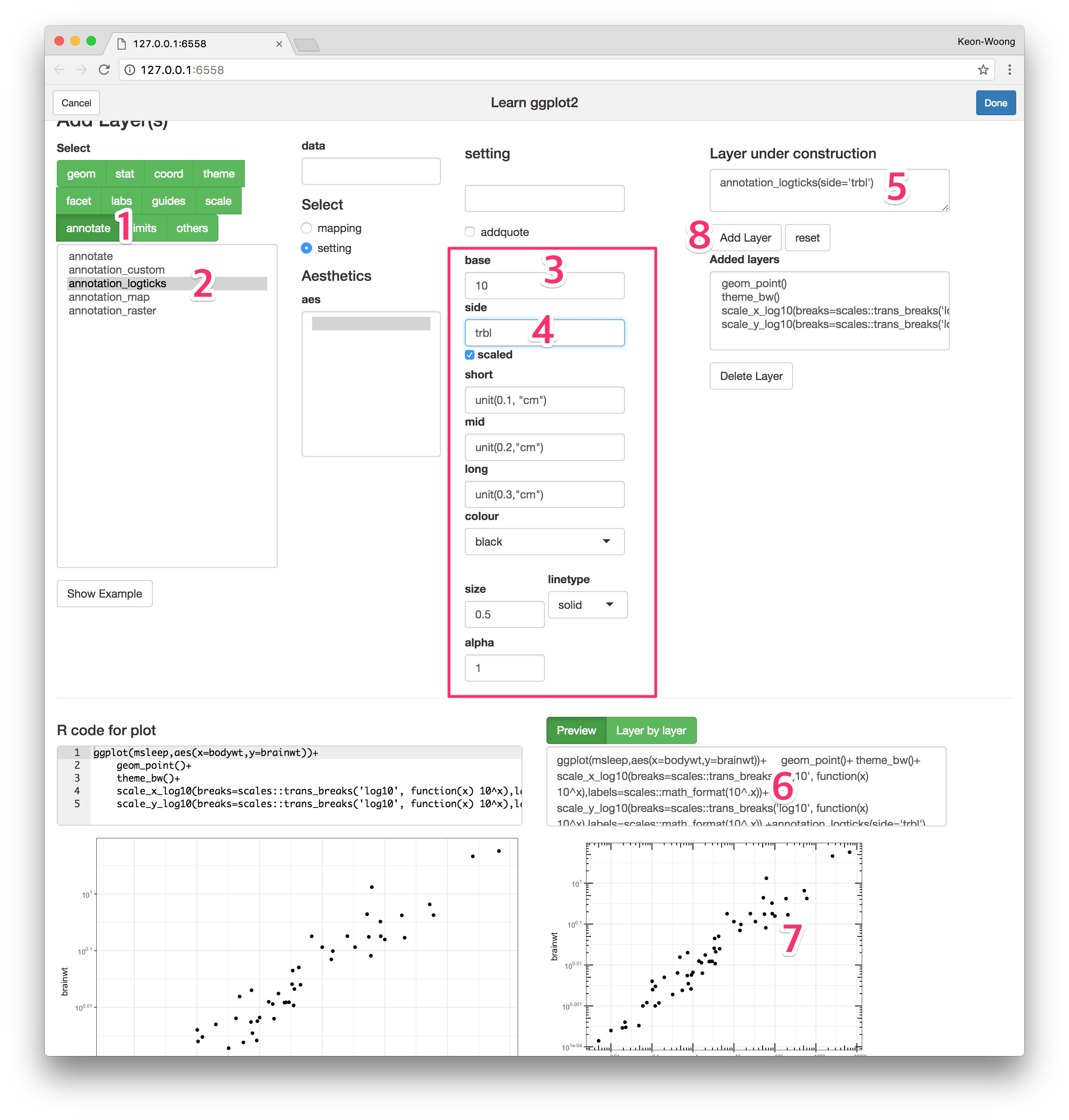1. You can hide the minor grid lines because they don’t align with the ticks. Press `theme` button(1) and select the `theme` function(2). The `theme()` function has a lot of arguments. Select `panel.grid.minor` argument(3) and select `element_blank()`(4) to hide the minor grid line.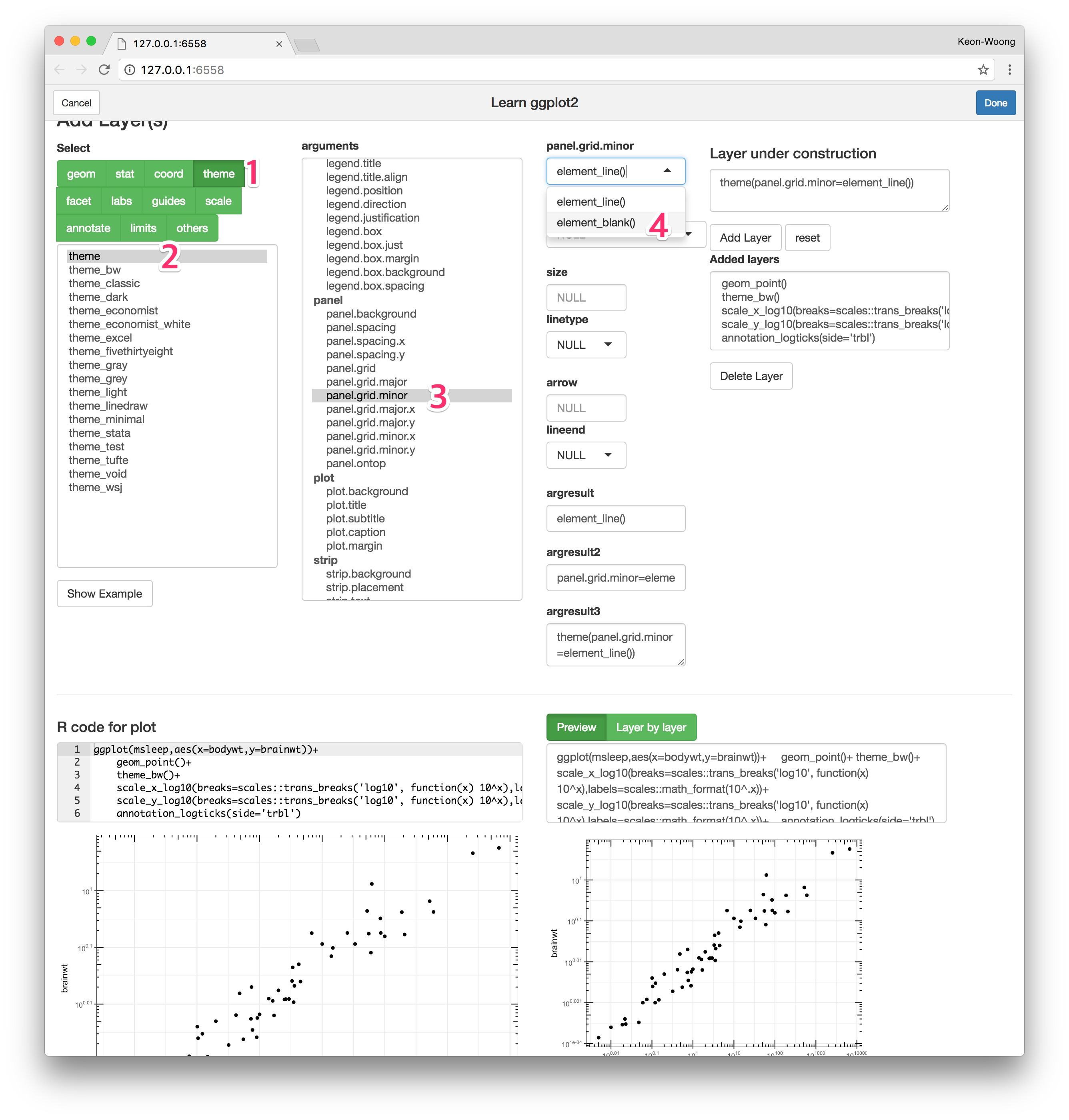1. You can see this plot layer by layer. Press the `Layer by layer` button(1) and use the sliderInput to see the plot layer by layer. You can animate this plot by click the arrowhead(2). If you want to get this R code for plot, press `Done` button(3).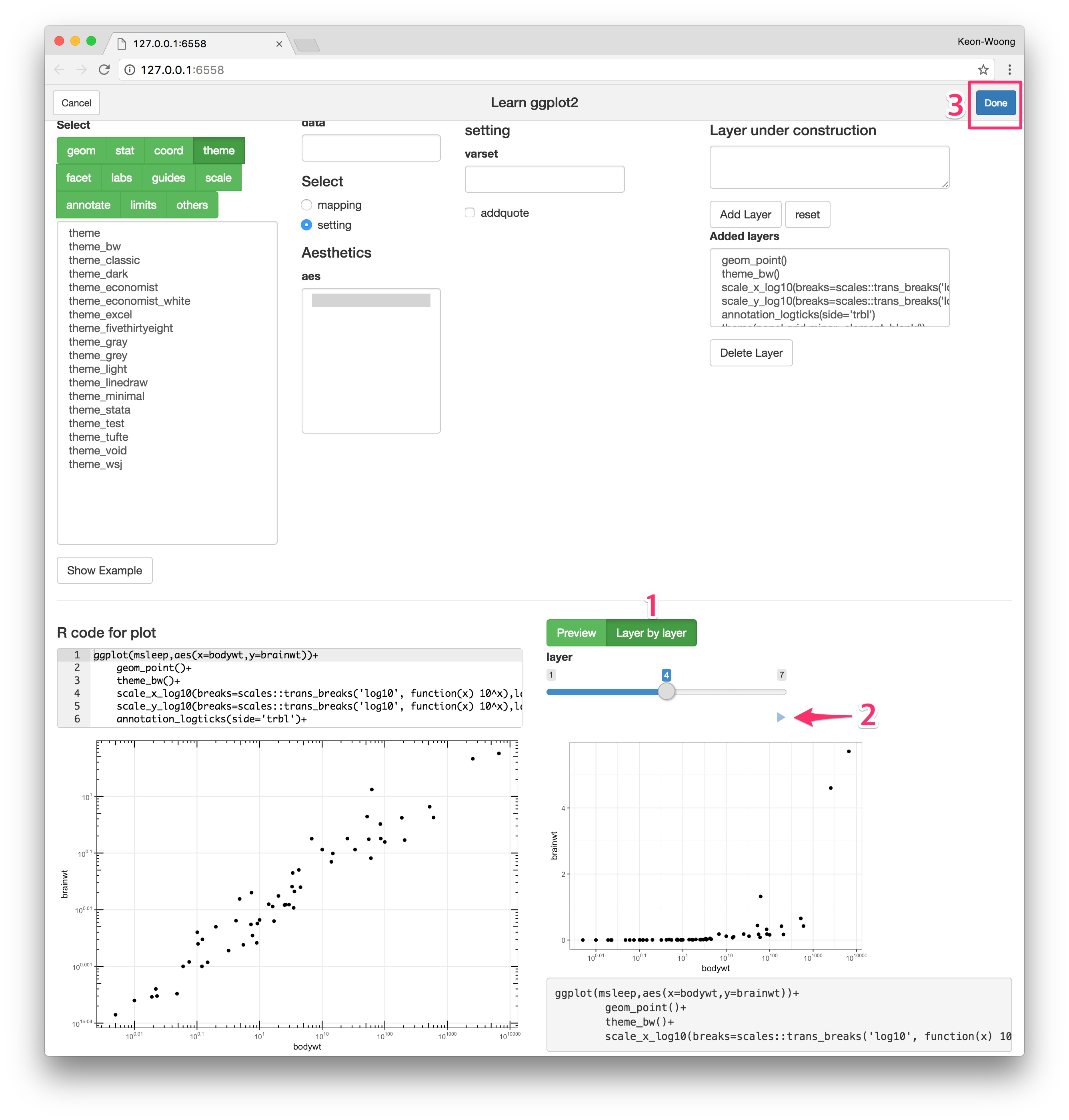1. When you’re done, the code for the ggplot will be emitted at the cursor position(scarlet rectangle).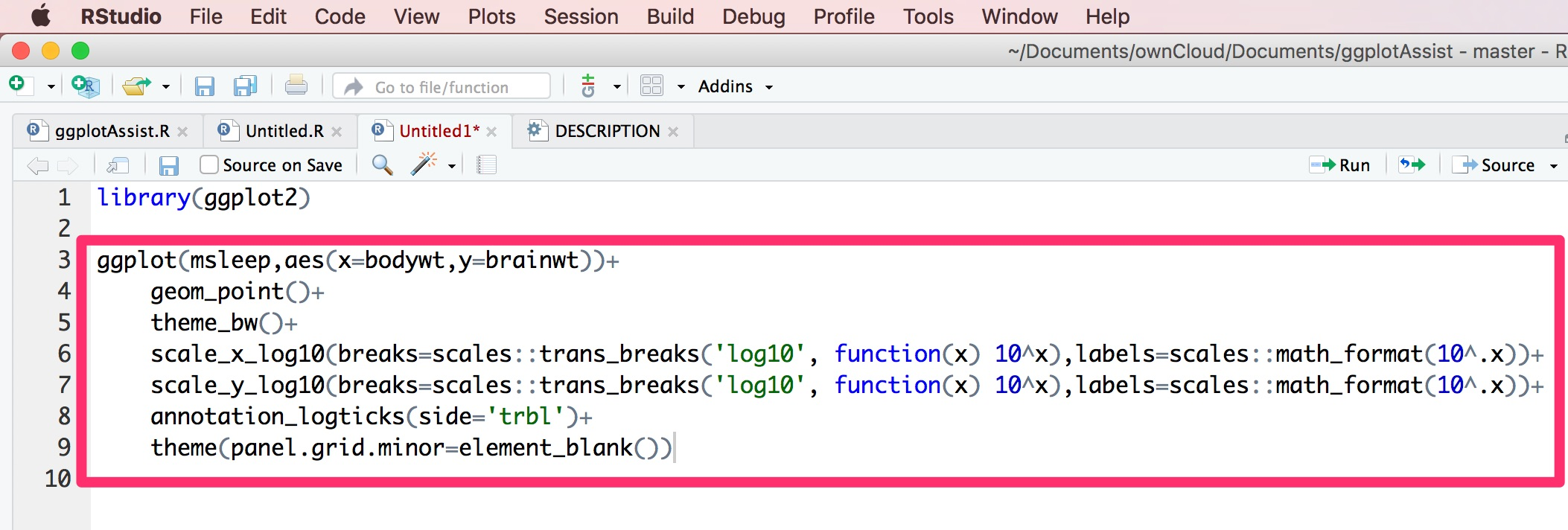## Usage: As a regular function

You can use the `ggplotAssist()` function as a regular function, e.g. in a command line.

``result <- ggplotAssist(mtcars)``

# Other topics

## How to use guide_colorbar()?

http://rpubs.com/cardiomoon/322415

## Recursive shiny module for functionals

There are many functions that takes a function as an input : Functionals. To handle a functional in a shiny app, you have to make a shiny module that allows recursive call. I have included an recursive shiny module `textFunction` in my package ggplotAssist. The UI of textFunction shiny module is `textFunctionInput` and the server function is `textFunction`. I also included two toy shiny app to demonstrate the recursive shiny module.

You can read the full story at https://github.com/cardiomoon/ggplotAssist/blob/master/textFunctionInput.md